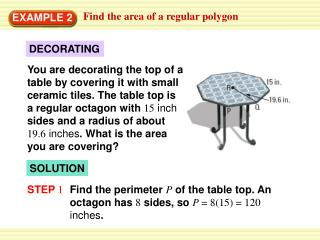DownloadDownload PresentationEXAMPLE 2

# EXAMPLE 2

Download Presentation## EXAMPLE 2

- - - - - - - - - - - - - - - - - - - - - - - - - - - E N D - - - - - - - - - - - - - - - - - - - - - - - - - - -
##### Presentation Transcript

1. You are decorating the top of a table by covering it with small ceramic tiles. The table top is a regular octagon with 15inch sides and a radius of about 19.6inches. What is the area you are covering? Find the area of a regular polygon EXAMPLE 2 DECORATING SOLUTION STEP 1 Find the perimeter Pof the table top. An octagon has 8 sides, so P = 8(15) = 120inches.

2. Find the apothem a. The apothem is height RSof ∆PQR. Because ∆PQRis isosceles, altitude RSbisects QP. 1 1 So,QS = (QP) = (15) = 7.5 inches. 2 2 √ a = RS ≈ √19.62 – 7.52 = 327.91 ≈ 18.108 Find the area of a regular polygon EXAMPLE 2 STEP 2 To find RS, use the Pythagorean Theorem for ∆ RQS.

3. 1 A = aP 2 1 ≈ (18.108)(120) 2 ANSWER So, the area you are covering with tiles is about 1086.5square inches. Find the area of a regular polygon EXAMPLE 2 STEP 3 Find the area Aof the table top. Formula for area of regular polygon Substitute. ≈ 1086.5 Simplify.

4. A regular nonagon is inscribed in a circle with radius 4 units. Find the perimeter and area of the nonagon. SOLUTION 360° The measure of central JLKis , or 40°. Apothem LMbisects the central angle, so m KLMis 20°. To find the lengths of the legs, use trigonometric ratios for right ∆KLM. 9 Find the perimeter and area of a regular polygon EXAMPLE 3

5. LM MK sin 20° = cos 20° = LK LK MK LM cos 20° = sin 20° = 4 4 4 sin 20° = MK 4 cos 20° = LM The regular nonagon has side length s = 2MK = 2(4 sin 20°) = 8  sin 20° and apothem a = LM = 4  cos20°. Find the perimeter and area of a regular polygon EXAMPLE 3

6. ANSWER So, the perimeter is P = 9s = 9(8 sin 20°) = 72 sin 20° ≈ 24.6 units, and the area is A = aP = (4 cos 20°)(72 sin20°)≈46.3 square units. 1 1 2 2 Find the perimeter and area of a regular polygon EXAMPLE 3

7. 3. ANSWER about 46.6 units, about 151.5 units2 for Examples 2 and 3 GUIDED PRACTICE Find the perimeter and the area of the regular polygon.

8. 4. ANSWER 70 units, about 377.0 units2 for Examples 2 and 3 GUIDED PRACTICE Find the perimeter and the area of the regular polygon.

9. 5. 30 3  52.0units, about 129.9 units2 ANSWER for Examples 2 and 3 GUIDED PRACTICE Find the perimeter and the area of the regular polygon.

10. ANSWER Exercise 5 for Examples 2 and 3 GUIDED PRACTICE 6. Which of Exercises 3–5 above can be solved using special right triangles?